# beta decay

(redirected from beta process)
Also found in: Dictionary, Thesaurus, Medical.

## beta decay

A type of radioactive decay in which an atomic nucleus spontaneously transforms into a daughter nucleus and either an electron plus antineutrino or a positron plus neutrino. The daughter nucleus has the same mass number as the parent nucleus but differs in atomic number by one. The electrons or positrons ejected by beta decay have a spread of energies, extra energy being taken up by the antineutrinos or neutrinos, respectively.
Collins Dictionary of Astronomy © Market House Books Ltd, 2006
The following article is from The Great Soviet Encyclopedia (1979). It might be outdated or ideologically biased.

## Beta Decay

β-decay, radioactive decay of an atomic nucleus accompanied by the escape of an electron or positron from the nucleus. This process is caused by a spontaneous transformation of one of the nucleons in the nucleus into a nucleon of another type—specifically, a transformation either of a neutron (n) into a proton (p) or of a proton into a neutron. In the former case, with an electron (e+) escaping from the nucleus, so-called β -decay takes place. In the latter case, with a positron (e+) escaping from the nucleus, β + -decay takes place. The electrons and positrons emitted in beta decay are termed beta particles. The mutual transformations of the nucleons are accompanied by the appearance of still another particle—the neutrino (v) in the case of β+ -decay, the antineutrino (v) in the case of β- -decay. In β- -decay, the number of protons (Z) in the nucleus increases by a unit and the number of neutrons decreases by a unit. The mass number A of the nucleus—equal to the total number of nucleons present in the nucleus—does not vary, and the product nucleus is an isobar of the original nucleus, standing on the right of the latter in the periodic table of the elements. Conversely, the number of protons in β+ -decay decreases by a unit and the number of neutrons increases by a unit, so that an isobar standing to the left of the original nucleus is formed. The two beta decay processes are written symbolically as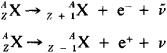where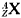is the symbol of the nucleus, consisting of Z protons and A - Z neutrons.

The simplest example of β- -decay is the transformation of a free neutron into a proton with the emission of an electron and an antineutrino (neutron half-life ≈ 13 min)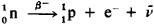A more involved example of β- -decay is the decay of a heavy hydrogen isotope, tritium, consisting of two neutrons (n) and one proton (p)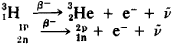Clearly, this process reduces to the β- -decay of a bound (nuclear) neutron. In that case the beta-radioactive tritium nucleus is transformed into the nucleus of the next element in the periodic table of elements—the nucleus of the light isotopeHe.

An example of β+ -decay is the decay of the carbon isotope 11C according to the schema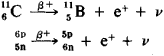This process can be represented as the decay of a bound proton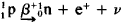. In this case the carbon nucleus is transformed into the nucleus of the preceding element in the periodic table—boron.

The transformation of a proton into a neutron within the nucleus can occur as the result of capture by the proton of one of the electrons from the electron shell of the atom. Capture of the electron e-k from the K-shell nearest the nucleus occurs most often and is termed K-capture. In K-capture, as in β+ -decay, an isobar standing to the left of the initial nucleus in the periodic table of the elements forms. The K-capture equation exhibits the form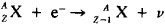After the K-electron has been captured, the place left unoccupied draws an electron from a higher shell, its place is occupied by an electron from a still higher shell, and so on; this results in the emission of photons. Hence, K-capture is accompanied by emission of characteristic X-radiation. One example of K-capture is the reaction in which the nucleus of a beryllium isotope captures a K-electron and is thereby transformed into a lithium nucleus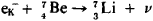Beta decay is observed in both naturally radioactive and artificially radioactive isotopes. In order for the nucleus to be unstable relative to one of the modes of β-transformation (that is, able to experience beta decay), the sum of the masses of the particles on the left side of the reaction equation must be greater than the sum of the masses of the transformation products. This accounts for the energy released in beta decay. The energy of beta decay Eβ can be calculated from this mass balance by utilizing the formula E = mc2, where c is the speed of light in a vacuum. In the case of β- -decay

Eβ-= (Mz - Mz+1,)c2

where M is the mass of the neutral atoms. In the case of β+ -decay, the neutral atom loses one of the electrons in its shell, and the beta-decay energy is

Eβ+ = (M2 - Mz-1 - 2me)c2

where me is the electron mass.

The energy of beta decay is distributed among three particles: the electron (or positron), the antineutrino (or neutrino), and the nucleus; therefore, beta particles are capable of carrying off practically any amount of energy from 0 to Eβ—that is, their energy spectra are continuous. In K-capture the neutrino always escapes with the same energy.

The investigation of the beta decay of nuclei has repeatedly presented scientists with unexpected dilemmas. After the discovery of radioactivity, the phenomenon of beta decay was long treated as an argument in favor of the presence of electrons within atomic nuclei; later, however, that assumption was found to be in clear contradiction to the findings of quantum mechanics. Then the diversity of the energy of the electrons escaping in beta decay inspired a lack of confidence in the law of conservation of energy, since it was known that nuclei in states of fully determined energy take part in that transformation. The suggestion by the German scientist W. Pauli of the existence of a new particle—the neutrino—saved not only the law of energy conservation but also another major law of physics—the law of conservation of angular momentum. Since the spins (that is, the intrinsic moments) of the neutron and proton are both ½ , spin conservation in the right-hand part of the beta-decay equations calls for an odd number of particles with spin of ½.

Beta decay is found in elements in all parts of the periodic table. The tendency to β-transformation is due to an excess of neutrons or protons in some isotopes over the amount corresponding to maximum stability. Hence, the tendency to β + -decay or to K-capture is typical of neutron-deficient isotopes, but the tendency to β- -decay is typical of neutron-excess isotopes. About 1,500 β -radioactive isotopes of all of the elements in the periodic table, except for the very heaviest (Z ≥ 102), are known. The energy of beta decay of presently known isotopes falls within a range from Eβ- = 0.0186 MeV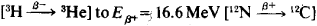the half-lives cover a broad range extending from 1.3 × 10–2 sec (12N) to ≈ 2 × 1013 years (the naturally occurring radioactive isotope 180W).

Both the lifetime of a nucleus with respect to beta decay and the shape of the beta-particle spectrum depend on the states in which the initial nucleon and the product nucleon are found within the nucleus. Hence, the study of beta decay not only yields information on the nature and properties of weak interactions but has also aided in formulating the concepts of the structure of atomic nuclei.

The probability of beta decay depends to a great extent on the proximity to each other of the states of the nucleons in the original and product nuclei. If the state of the nucleon undergoes no change, then the probability of beta decay is maximized, and the corresponding transition from the original state to the product state is said to be permitted. Such transitions are typical of beta decay of light nuclei. Light nuclei contain an almost identical number of neutrons and protons. In heavier nuclei, the number of neutrons exceeds the number of protons. The states of nucleons of different types differ substantially. This hinders beta decay; transitions occur in which beta decay takes place with low probability. Transition is also inhibited by the requirement that the spin of the nucleus change; these transitions are called forbidden transitions. The nature of the transition also has its effect on the shape of the energy spectrum of the beta particles.

Experimental investigation of the energy distribution of electrons emitted by beta-radioactive nuclei (beta spectra) is carried out with the aid of beta spectrometers. Examples of beta spectra are shown in Figures 1 and 2.

The nature of the interactions responsible for beta decay is still far from thoroughly investigated. This interaction is known as the weak interaction, since it is 1012 times weaker than the nuclear interaction and 109 times weaker than the electromagnetic interaction. (It exceeds only the gravitational interaction.) Weak interaction is inherent in all elementary particles except the photon. The breakdown of right-left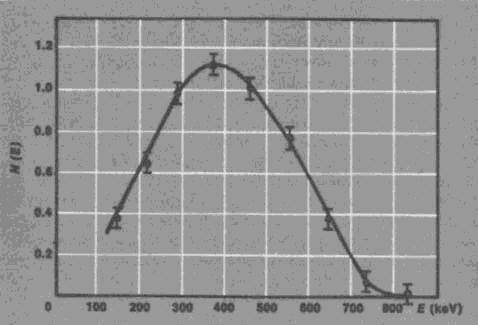Figure 1. Beta Spectrum of a neutron. The kinetic energy E of the electrons (in keV) is shown on the horizontal axis; the number of electrons N (E) in relative units is shown on the vertical axis. (The limits of error of measurement of electrons with given energy are indicated by the vertical lines.)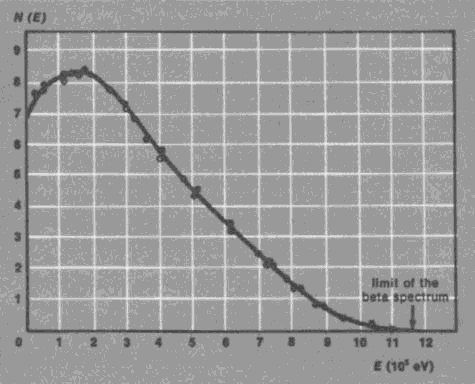Figure 2. Beta spectrum of RaE (example of beta spectrum of a heavy element)

symmetry was discovered in the study of beta decay. This nonconservation of spatial parity was found to be the property of weak interactions.

### REFERENCES

Al’fa-, beta-igamma-spektroskopiia. Edited by K. Siegbahn. Moscow, 1969. Chapters 22–24. (Translated from English.)
Eksperimental’naia iadernaia fizika, vol. 3. Edited by E. Segrè. Moscow, 1961. (Translated from English.)

E. M. LEIKIN [3–817–2; updated]